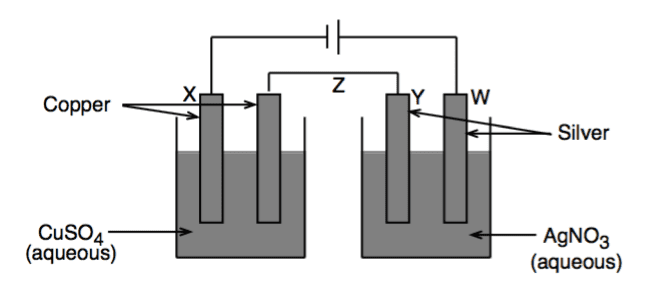# Galvanic cell changes

## Homework Statement

During electrolysis in the cell below, 1.05 g copper is deposited on electrode X. Which one of the following statements is correct?

(Relative atomic masses Cu = 63, Ag = 108).1. A) The mass of silver electrode W decreases by 3.60 g.

2. B) The mass of silver deposited on electrode Y during the electrolysis is 1.80 g.

3. C) Z is a salt-bridge.

4. D) The concentration of copper ions in solution decreases during the electrolysis.

5. E) The flow of negative charge is from right to left in Z.

## The Attempt at a Solution

I chose A. Since 1.05g of Cu is deposited, there are 0.01667 mol of Cu deposited. This means there are 0.0333 mol of electrons transferred. Which means there are 0.0333 mol of Ag lost in the anode. This means the anode lost 3.60g of Ag. However, C also makes sense as Z is the salt bridge. Could someone tell me where I went wrong?

•